8种主要排序算法的C#实现

PS：有可能实现的代码并不是最优的，如果有什么错误或者值得改进的地方，还请大家帮忙指出。

简介

平均时间复杂度从高到低依次是：

冒泡排序（o(n2)），选择排序（o(n2)），插入排序（o(n2)），堆排序（o(nlogn)），

归并排序（o(nlogn)），快速排序（o(nlogn)）， 希尔排序（o(n1.25)），基数排序（o(n)）

List<int>有ToArray()，哈哈！)。

选择排序

1         public static void SelectSort(IList<int> data)
2         {
3             for (int i = 0; i < data.Count - 1; i++)
4             {
5                 int min = i;
6                 int temp = data[i];
7                 for (int j = i + 1; j < data.Count; j++)
8                 {
9                     if (data[j] < temp)
10                     {
11                         min = j;
12                         temp = data[j];
13                     }
14                 }
15                 if (min != i)
16                     Swap(data, min, i);
17             }
18         }

冒泡排序

1         public static void BubbleSort(IList<int> data)
2         {
3             for (int i = data.Count - 1; i > 0; i--)
4             {
5                 for (int j = 0; j < i; j++)
6                 {
7                     if (data[j] > data[j + 1])
8                         Swap(data, j, j + 1);
9                 }
10             }
11         }

通过标识提升冒泡排序

1         public static void BubbleSortImprovedWithFlag(IList<int> data)
2         {
3             bool flag;
4             for (int i = data.Count - 1; i > 0; i--)
5             {
6                 flag = true;
7                 for (int j = 0; j < i; j++)
8                 {
9                     if (data[j] > data[j + 1])
10                     {
11                         Swap(data, j, j + 1);
12                         flag = false;
13                     }
14                 }
15                 if (flag) break;
16             }
17         }

鸡尾酒排序（来回排序）

对冒泡排序进行更大的优化

1         public static void BubbleCocktailSort(IList<int> data)
2         {
3             bool flag;
4             int m = 0, n = 0;
5             for (int i = data.Count - 1; i > 0; i--)
6             {
7                 flag = true;
8                 if (i % 2 == 0)
9                 {
10                     for (int j = n; j < data.Count - 1 - m; j++)
11                     {
12                         if (data[j] > data[j + 1])
13                         {
14                             Swap(data, j, j + 1);
15                             flag = false;
16                         }
17                     }
18                     if (flag) break;
19                     m++;
20                 }
21                 else
22                 {
23                     for (int k = data.Count - 1 - m; k > n; k--)
24                     {
25                         if (data[k] < data[k - 1])
26                         {
27                             Swap(data, k, k - 1);
28                             flag = false;
29                         }
30                     }
31                     if (flag) break;
32                     n++;
33                 }
34             }
35         }

插入排序

1         public static void InsertSort(IList<int> data)
2         {
3             int temp;
4             for (int i = 1; i < data.Count; i++)
5             {
6                 temp = data[i];
7                 for (int j = i - 1; j >= 0; j--)
8                 {
9                     if (data[j] > temp)
10                     {
11                         data[j + 1] = data[j];
12                         if (j == 0)
13                         {
14                             data = temp;
15                             break;
16                         }
17                     }
18                     else
19                     {
20                         data[j + 1] = temp;
21                         break;
22                     }
23                 }
24             }
25         }

二分查找法优化插入排序

1         public static void InsertSortImprovedWithBinarySearch(IList<int> data)
2         {
3             int temp;
4             int tempIndex;
5             for (int i = 1; i < data.Count; i++)
6             {
7                 temp = data[i];
8                 tempIndex = BinarySearchForInsertSort(data, 0, i, i);
9                 for (int j = i - 1; j >= tempIndex; j--)
10                 {
11                     data[j + 1] = data[j];
12                 }
13                 data[tempIndex] = temp;
14             }
15         }
16
17         public static int BinarySearchForInsertSort(IList<int> data, int low, int high, int key)
18         {
19             if (low >= data.Count - 1)
20                 return data.Count - 1;
21             if (high <= 0)
22                 return 0;
23             int mid = (low + high) / 2;
24             if (mid == key) return mid;
25             if (data[key] > data[mid])
26             {
27                 if (data[key] < data[mid + 1])
28                     return mid + 1;
29                 return BinarySearchForInsertSort(data, mid + 1, high, key);
30             }
31             else  // data[key] <= data[mid]
32             {
33                 if (mid - 1 < 0) return 0;
34                 if (data[key] > data[mid - 1])
35                     return mid;
36                 return BinarySearchForInsertSort(data, low, mid - 1, key);
37             }
38         }

过程解析：需要注意的是二分查找方法实现中high-low==1的时候mid==low，所以需要33行

mid-1<0即mid==0的判断，否则下行会索引越界。

快速排序

1         public static void QuickSortStrict(IList<int> data)
2         {
3             QuickSortStrict(data, 0, data.Count - 1);
4         }
5
6         public static void QuickSortStrict(IList<int> data, int low, int high)
7         {
8             if (low >= high) return;
9             int temp = data[low];
10             int i = low + 1, j = high;
11             while (true)
12             {
13                 while (data[j] > temp) j--;
14                 while (data[i] < temp && i < j) i++;
15                 if (i >= j) break;
16                 Swap(data, i, j);
17                 i++; j--;
18             }
19             if (j != low)
20                 Swap(data, low, j);
21             QuickSortStrict(data, j + 1, high);
22             QuickSortStrict(data, low, j - 1);
23         }

另一个版本

1         public static void QuickSortRelax(IList<int> data)
2         {
3             QuickSortRelax(data, 0, data.Count - 1);
4         }
5
6         public static void QuickSortRelax(IList<int> data, int low, int high)
7         {
8             if (low >= high) return;
9             int temp = data[(low + high) / 2];
10             int i = low - 1, j = high + 1;
11             while (true)
12             {
13                 while (data[++i] < temp) ;
14                 while (data[--j] > temp) ;
15                 if (i >= j) break;
16                 Swap(data, i, j);
17             }
18             QuickSortRelax(data, j + 1, high);
19             QuickSortRelax(data, low, i - 1);
20         }

针对这个版本的缺点，我进行了优化

1         public static void QuickSortRelaxImproved(IList<int> data)
2         {
3             QuickSortRelaxImproved(data, 0, data.Count - 1);
4         }
5
6         public static void QuickSortRelaxImproved(IList<int> data, int low, int high)
7         {
8             if (low >= high) return;
9             int temp = data[(low + high) / 2];
10             int i = low - 1, j = high + 1;
11             int index = (low + high) / 2;
12             while (true)
13             {
14                 while (data[++i] < temp) ;
15                 while (data[--j] > temp) ;
16                 if (i >= j) break;
17                 Swap(data, i, j);
18                 if (i == index) index = j;
19                 else if (j == index) index = i;
20             }
21             if (j == i)
22             {
23                 QuickSortRelaxImproved(data, j + 1, high);
24                 QuickSortRelaxImproved(data, low, i - 1);
25             }
26             else //i-j==1
27             {
28                 if (index >= i)
29                 {
30                     if (index != i)
31                         Swap(data, index, i);
32                     QuickSortRelaxImproved(data, i + 1, high);
33                     QuickSortRelaxImproved(data, low, i - 1);
34                 }
35                 else //index < i
36                 {
37                     if (index != j)
38                         Swap(data, index, j);
39                     QuickSortRelaxImproved(data, j + 1, high);
40                     QuickSortRelaxImproved(data, low, j - 1);
41                 }
42             }
43         }

归并排序

1         public static List<int> MergeSortOnlyList(List<int> data, int low, int high)
2         {
3             if (low == high)
4                 return new List<int> { data[low] };
5             List<int> mergeData = new List<int>();
6             int mid = (low + high) / 2;
7             List<int> leftData = MergeSortOnlyList(data, low, mid);
8             List<int> rightData = MergeSortOnlyList(data, mid + 1, high);
9             int i = 0, j = 0;
10             while (true)
11             {
12                 if (leftData[i] < rightData[j])
13                 {
15                     if (++i == leftData.Count)
16                     {
18                         break;
19                     }
20                 }
21                 else
22                 {
24                     if (++j == rightData.Count)
25                     {
27                         break;
28                     }
29                 }
30             }
31             return mergeData;
32         }
33
34         public static List<int> MergeSortOnlyList(List<int> data)
35         {
36             data = MergeSortOnlyList(data, 0, data.Count - 1);  //不会改变外部引用 参照C#参数传递
37             return data;
38         }

IList<int>版本

1         public static IList<int> MergeSort(IList<int> data)
2         {
3             data = MergeSort(data, 0, data.Count - 1);
4             return data;
5         }
6
7         public static IList<int> MergeSort(IList<int> data, int low, int high)
8         {
9             int length = high - low + 1;
10             IList<int> mergeData = NewInstance(data, length);
11             if (low == high)
12             {
13                 mergeData = data[low];
14                 return mergeData;
15             }
16             int mid = (low + high) / 2;
17             IList<int> leftData = MergeSort(data, low, mid);
18             IList<int> rightData = MergeSort(data, mid + 1, high);
19             int i = 0, j = 0;
20             while (true)
21             {
22                 if (leftData[i] < rightData[j])
23                 {
24                     mergeData[i + j] = leftData[i++]; //不能使用Add,Array Length不可变
25                     if (i == leftData.Count)
26                     {
27                         int rightLeft = rightData.Count - j;
28                         for (int m = 0; m < rightLeft; m++)
29                         {
30                             mergeData[i + j] = rightData[j++];
31                         }
32                         break;
33                     }
34                 }
35                 else
36                 {
37                     mergeData[i + j] = rightData[j++];
38                     if (j == rightData.Count)
39                     {
40                         int leftleft = leftData.Count - i;
41                         for (int n = 0; n < leftleft; n++)
42                         {
43                             mergeData[i + j] = leftData[i++];
44                         }
45                         break;
46                     }
47                 }
48             }
49             return mergeData;
50
51         }

堆排序

1         public static void HeapSort(IList<int> data)
2         {
3             BuildMaxHeapify(data);
4             int j = data.Count;
5             for (int i = 0; i < j; )
6             {
7                 Swap(data, i, --j);
8                 if (j - 2 < 0)  //只剩下1个数 j代表余下要排列的数的个数
9                     break;
10                 int k = 0;
11                 while (true)
12                 {
13                     if (k > (j - 2) / 2) break;  //即：k > ((j-1)-1)/2 超出最后一个父节点的位置
14                     else
15                     {
16                         int temp = k;
17                         k = ReSortMaxBranch(data, k, 2 * k + 1, 2 * k + 2, j - 1);
18                         if (temp == k) break;
19                     }
20                 }
21             }
22         }
23
24         public static void BuildMaxHeapify(IList<int> data)
25         {
26             for (int i = data.Count / 2 - 1; i >= 0; i--)  //(data.Count-1)-1)/2为数列最大父节点索引
27             {
28                 int temp = i;
29                 temp = ReSortMaxBranch(data, i, 2 * i + 1, 2 * i + 2, data.Count - 1);
30                 if (temp != i)
31                 {
32                     int k = i;
33                     while (k != temp && temp <= data.Count / 2 - 1)
34                     {
35                         k = temp;
36                         temp = ReSortMaxBranch(data, temp, 2 * temp + 1, 2 * temp + 2, data.Count - 1);
37                     }
38                 }
39             }
40         }
41
42         public static int ReSortMaxBranch(IList<int> data, int maxIndex, int left, int right, int lastIndex)
43         {
44             int temp;
45             if (right > lastIndex)  //父节点只有一个子节点
46                 temp = left;
47             else
48             {
49                 if (data[left] > data[right])
50                     temp = left;
51                 else temp = right;
52             }
53
54             if (data[maxIndex] < data[temp])
55                 Swap(data, maxIndex, temp);
56             else temp = maxIndex;
57             return temp;
58         }

（即父节点与它的两个子节点）符合父节点值最大的规则。ReSortMaxBranch为将三角调整为父节点值最大，

希尔排序

1         public static void ShellSort(IList<int> data)
2         {
3             int temp;
4             for (int gap = data.Count / 2; gap > 0; gap /= 2)
5             {
6                 for (int i = gap; i < data.Count; i += gap)
7                 {
8                     temp = data[i];
9                     for (int j = i - gap; j >= 0; j -= gap)
10                     {
11                         if (data[j] > temp)
12                         {
13                             data[j + gap] = data[j];
14                             if (j == 0)
15                             {
16                                 data[j] = temp;
17                                 break;
18                             }
19                         }
20                         else
21                         {
22                             data[j + gap] = temp;
23                             break;
24                         }
25                     }
26                 }
27             }
28         }

——————

1         public static void ShellSortCorrect(IList<int> data)
2         {
3             int temp;
4             for (int gap = data.Count / 2; gap > 0; gap /= 2)
5             {
6                 for (int i = gap; i < data.Count; i++)      // i+ = gap 改为了 i++
7                 {
8                     temp = data[i];
9                     for (int j = i - gap; j >= 0; j -= gap)
10                     {
11                         if (data[j] > temp)
12                         {
13                             data[j + gap] = data[j];
14                             if (j == 0)
15                             {
16                                 data[j] = temp;
17                                 break;
18                             }
19                         }
20                         else
21                         {
22                             data[j + gap] = temp;
23                             break;
24                         }
25                     }
26                 }
27             }
28         }——————

基数排序

“非比较型”是什么意思呢？因为它内部使用的是桶排序，而桶排序是非比较型排序。

桶排序

1，9999的桶排序实现：new Int;总共有两个数，得出最大数9999的位数4，取10的4次幂即10000作为分母，

为基数排序做个过渡，我这里实现了一个个位数桶排序

1         public static void BucketSortOnlyUnitDigit(IList<int> data)
2         {
3             int[] indexCounter = new int;
4             for (int i = 0; i < data.Count; i++)
5             {
6                 indexCounter[data[i]]++;
7             }
8             int[] indexBegin = new int;
9             for (int i = 1; i < 10; i++)
10             {
11                 indexBegin[i] = indexBegin[i-1]+ indexCounter[i-1];
15             }
16             IList<int> tempList = NewInstance(data, data.Count);
17             for (int i = 0; i < data.Count; i++)
18             {
19                 int number = data[i];
20                 tempList[indexBegin[number]++] = data[i];
21             }
22             data = tempList;
23         }

0,0,2。当1个1已取出排序，那索引将+1,变为0,1,2。这样就通过提前给重复的数空出位置，解决了

1         public static IList<int> RadixSort(IList<int> data)
2         {
3             int max = data;
4             for (int i = 1; i < data.Count; i++)
5             {
6                 if (data[i] > max)
7                     max = data[i];
8             }
9             int digit = 1;
10             while (max / 10 != 0)
11             {
12                 digit++;
13                 max /= 10;
14             }
15             for (int i = 0; i < digit; i++)
16             {
17                 int[] indexCounter = new int;
18                 IList<int> tempList = NewInstance(data, data.Count);
19                 for (int j = 0; j < data.Count; j++)
20                 {
21                     int number = (data[j] % Convert.ToInt32(Math.Pow(10, i + 1))) / Convert.ToInt32(Math.Pow(10, i));  //得出i+1位上的数
22                     indexCounter[number]++;
23                 }
24                 int[] indexBegin = new int;
25                 for (int k = 1; k < 10; k++)
26                 {
27                     indexBegin[k] = indexBegin[k - 1] + indexCounter[k - 1];
28                 }
29                 for (int k = 0; k < data.Count; k++)
30                 {
31                     int number = (data[k] % Convert.ToInt32(Math.Pow(10, i + 1))) / Convert.ToInt32(Math.Pow(10, i));
32                     tempList[indexBegin[number]++] = data[k];
33                 }
34                 data = tempList;
35             }
36             return data;
37         }

测试比较

1         public static int[] RandomSet(int length, int max)
2         {
3             int[] result = new int[length];
4             Random rand = new Random();
5             for (int i = 0; i < result.Length; i++)
6             {
7                 result[i] = rand.Next(max);
8             }
9             return result;
10         }
11
12         public static bool IsAscOrdered(IList<int> data)
13         {
14             bool flag = true;
15             for (int i = 0; i < data.Count - 1; i++)
16             {
17                 if (data[i] > data[i + 1])
18                     flag = false;
19             }
20             return flag;
21         }
22
23         public static void TestMicrosoft(IList<int> data)
24         {
25             Stopwatch stopwatch = new Stopwatch();
26             stopwatch.Start();
27             List<int> result = data.OrderBy(a => a).ToList();
28             stopwatch.Stop();
29             string methodName = "TestMicrosoft";
30             int length = methodName.Length;
31             for (int i = 0; i < 40 - length; i++)
32             {
33                 methodName += " ";
34             }
35             Console.WriteLine(methodName +
36                 "  IsAscOrdered:" + IsAscOrdered(result) + "  Time:" + stopwatch.Elapsed.TotalSeconds);
37
38         }

1         static void Main(string[] args)
2         {
3             int[] aa = RandomSet(50000, 99999);
4             //int[] aa = OrderedSet(5000);
5             Console.WriteLine("Array Length:" + aa.Length);
6             RunTheMethod((Action<IList<int>>)SelectSort, aa.Clone() as int[]);
7             RunTheMethod((Action<IList<int>>)BubbleSort, aa.Clone() as int[]);
8             RunTheMethod((Action<IList<int>>)BubbleSortImprovedWithFlag, aa.Clone() as int[]);
9             RunTheMethod((Action<IList<int>>)BubbleCocktailSort, aa.Clone() as int[]);
10             RunTheMethod((Action<IList<int>>)InsertSort, aa.Clone() as int[]);
11             RunTheMethod((Action<IList<int>>)InsertSortImprovedWithBinarySearch, aa.Clone() as int[]);
12             RunTheMethod((Action<IList<int>>)QuickSortStrict, aa.Clone() as int[]);
13             RunTheMethod((Action<IList<int>>)QuickSortRelax, aa.Clone() as int[]);
14             RunTheMethod((Action<IList<int>>)QuickSortRelaxImproved, aa.Clone() as int[]);
15             RunTheMethod((Func<IList<int>, IList<int>>)MergeSort, aa.Clone() as int[]);
16             RunTheMethod((Action<IList<int>>)ShellSort, aa.Clone() as int[]);
17             RunTheMethod((Func<IList<int>, IList<int>>)RadixSort, aa.Clone() as int[]);
18             RunTheMethod((Action<IList<int>>)HeapSort, aa.Clone() as int[]);
19             TestMicrosoft(aa.Clone() as int[]);
21         }
22
23         public static void RunTheMethod(Func<IList<int>, IList<int>> method, IList<int> data)
24         {
25             Stopwatch stopwatch = new Stopwatch();
26             stopwatch.Start();
27             IList<int> result = method(data);
28             stopwatch.Stop();
29             string methodName = method.Method.Name;
30             int length = methodName.Length;
31             for (int i = 0; i < 40 - length; i++)
32             {
33                 methodName += " ";
34             }
35             Console.WriteLine(methodName +
36                 "  IsAscOrdered:" + IsAscOrdered(result) + "  Time:" + stopwatch.Elapsed.TotalSeconds);
37         }
38
39         public static void RunTheMethod(Action<IList<int>> method, IList<int> data)
40         {
41             Stopwatch stopwatch = new Stopwatch();
42             stopwatch.Start();
43             method(data);
44             stopwatch.Stop();
45             string methodName = method.Method.Name;
46             int length = methodName.Length;
47             for (int i = 0; i < 40 - length; i++)
48             {
49                 methodName += " ";
50             }
51             Console.WriteLine(methodName +
52                 "  IsAscOrdered:" + IsAscOrdered(data) + "  Time:" + stopwatch.Elapsed.TotalSeconds);
53         }public static void Swap(IList<int> data, int a, int b)
{
int temp = data[a];
data[a] = data[b];
data[b] = temp;
}

public static int[] OrderedSet(int length)
{
int[] result = new int[length];
for (int i = 0; i < length; i++)
{
result[i] = i;
}
return result;
}

public static IList<int> NewInstance(IList<int> data, int length)
{
IList<int> instance;
if (data is Array)
{
instance = new int[length];
}
else
{
instance = new List<int>(length);
for (int n = 0; n < length; n++)
{
}
}
return instance;
}
View Code

100000,50000,10000,5000,1000,100依次是：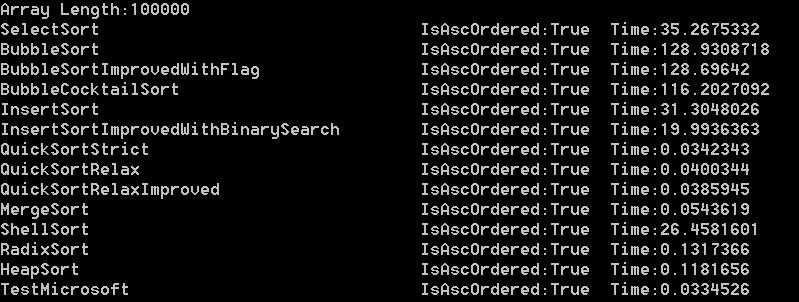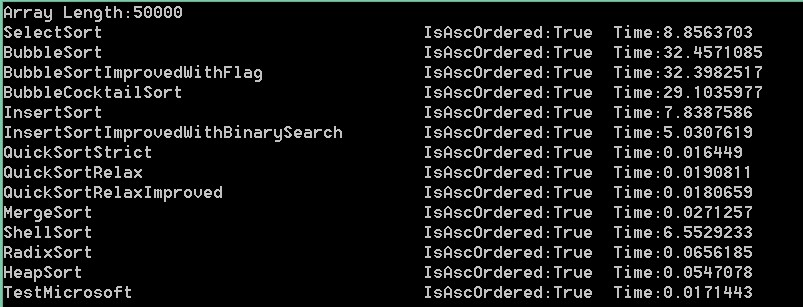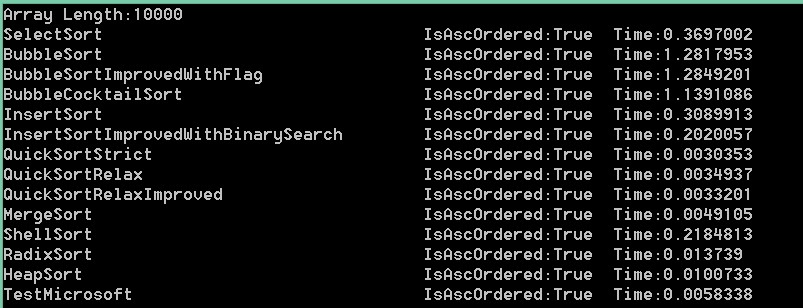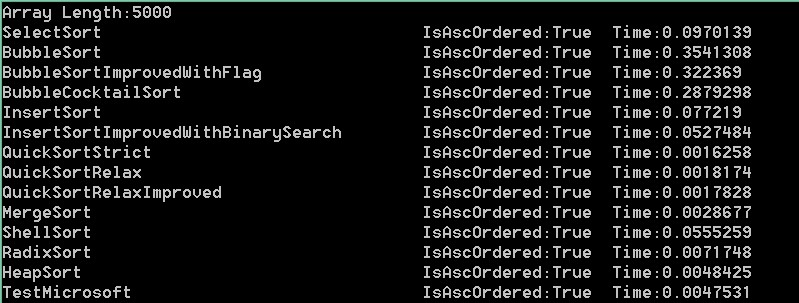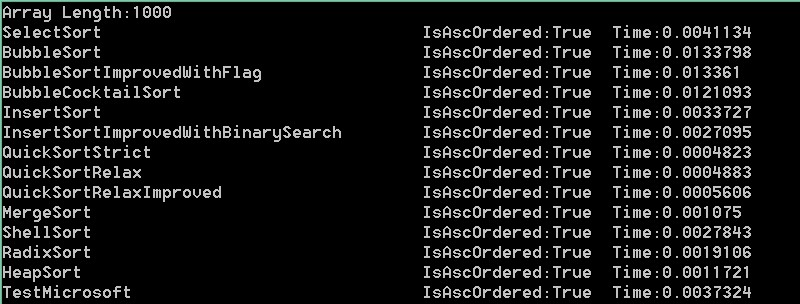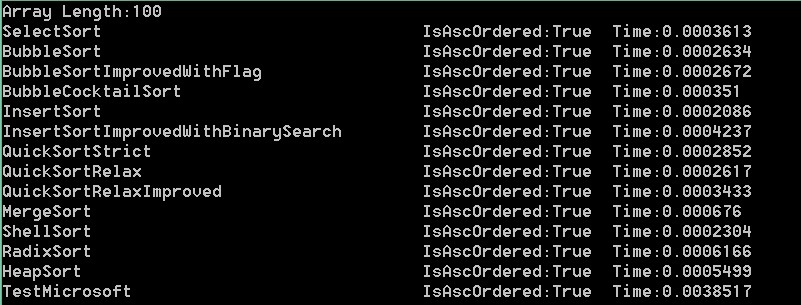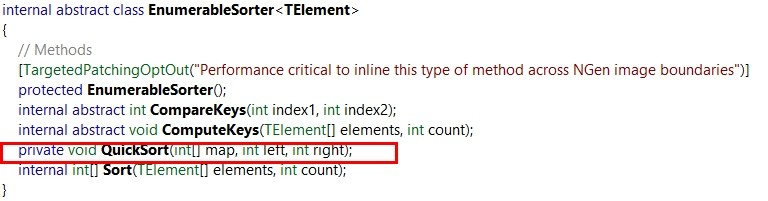总结

Demo源码

PS:我打算三月份去广州发展，主要会Asp.net mvc+jquery（不介意学习新的技术[除了webform]及语言[除了java]）。

posted @ 2014-01-23 14:02  胖鸟低飞  阅读(24723)  评论(38编辑  收藏  举报